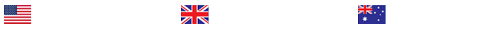Roto Air plans to spend \$1,000,000 on this project, resulting in a total savings of \$2,500,000 over the life of the project. Calculate the Benefit Cost Ratio.

Channels of Communication

It has been estimated that project managers spend up to 90% of their time communicating and your position at Roto Air is typical. Calculate the number of possible channels of communication if the project team were to grow to nine members, Important: show your work.
The total number of stakeholders, including the project team, is estimated at 75 people. Calculate how many more possible channels of communication the additional stakeholders beyond the team members represent. Important: show your work.
Benefit Cost Ratio (BCR)

Roto Air plans to spend \$1,000,000 on this project, resulting in a total savings of \$2,500,000 over the life of the project. Calculate the Benefit Cost Ratio. Important: show your work. Ignore any effects of the time value of money.
Roto Air also considered another project that would have partially satisfied their needs. It would have required a \$750,000 investment and would result in a savings of \$125,000 per year for 10 years. Calculate the Benefit Cost Ratio. Important: show your work. Ignore any effects of the time value of money.
Comparing only the BCRs, explain which project should Roto Air should have chosen. Ignore any effects of the time value of money.
Payback Period

Calculate the payback period for the \$1,000,000 investment shown above. Important: show your work. Ignore any effects of the time value of money.
Calculate the payback period for the \$750,000 investment shown above. Important: show your work. Ignore any effects of the time value of money.
Comparing only on the payback periods, explain which project should Roto Air have chosen. Ignore any effects of the time value of money.
Net Present Value (NPV)

Calculate the Net Present Value of Roto Air’s \$1,000,000 investment with a \$250,000 annual savings for 10 years when the interest rate averages 3% annually. Important: show your work.
Carefully review the Grading Rubric for the criteria that will be used to evaluate your assignment.

Description:

Total Possible Score: 10.00

Calculates the Correct Answers for Problems
Total: 6.00

Distinguished – Calculates the correct answers for all four problems.

Proficient – Calculates the correct answers for three of the problems.

Basic – Calculates the correct answers for two of the problems.

Below Expectations – Calculates the correct answer for one of the problems.

Non-Performance – The calculations are either nonexistent or lack the components described in the assignment instructions.

Utilizes a MS Excel Spreadsheet, Shows Work, and Provides Sources for the Equations Used
Total: 2.00

Distinguished – Utilizes a MS Excel spreadsheet, shows all work, and provides sources for the equations used.

Proficient – Utilizes a MS Excel spreadsheet, shows most work, and provides sources for some of the equations used.

Basic – Utilizes a MS Excel spreadsheet, shows some work, and provides sources for some of the equations used.

Below Expectations – Does not utilize a MS Excel spreadsheet, shows some work, and provides sources for some of the equations used.

Non-Performance – The spreadsheet, work, and sources are either nonexistent or lacks the components described in the assignment instructions.

Quantitative Literacy: Representation
Total: 1.00

Distinguished – Proficiently converts pertinent information into an insightful mathematical representation in a way that contributes to a further or deeper understanding.

Proficient – Competently converts pertinent information into an appropriate mathematical representation.

Basic – Converts information, but the outcomes are only partially appropriate or accurate.

Below Expectations – Attempts to convert information, but the outcomes are inappropriate or inaccurate.

Non-Performance – The assignment is either nonexistent or lacks the components described in the instructions.

Quantitative Literacy: Calculation
Total: 1.00

Distinguished – All calculations are correct and adequately all inclusive. Calculations are presented clearly and succinctly.

Proficient – Calculations are mostly accurate and adequately comprehensive to solve the problem.

Basic – Calculations are somewhat accurate and/or represent only a limited number of the calculations required to solve the problem.

Below Expectations – Calculations were attempted but are incorrect and are not comprehensive.

Non-Performance – The assignment is either nonexistent or lacks the components described in the instructions.

### Last Completed Projects

# topic title discipline academic level pages delivered
6
Writer's choice
University
2
1 hour 32 min
7
Wise Approach to
Philosophy
College
2
2 hours 19 min
8
1980's and 1990
History
College
3
2 hours 20 min
9
pick the best topic
Finance
School
2
2 hours 27 min
10
finance for leisure
Finance
University
12
2 hours 36 min